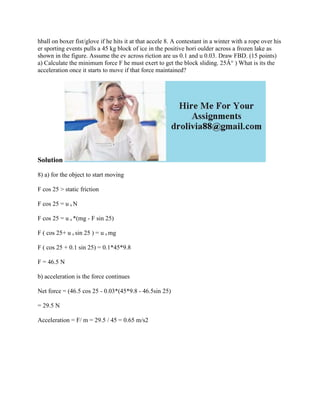Diese Präsentation wurde erfolgreich gemeldet.

# hball on boxer fist-glove if he hits it at that accele 8- A contestant.docx

Anzeige
Anzeige
Anzeige
Anzeige
Anzeige
Anzeige
Anzeige
Anzeige
Anzeige
Anzeige
Anzeige×

1 von 2 Anzeige

# hball on boxer fist-glove if he hits it at that accele 8- A contestant.docx

hball on boxer fist/glove if he hits it at that accele 8. A contestant in a winter with a rope over his er sporting events pulls a 45 kg block of ice in the positive hori oulder across a frozen lake as shown in the figure. Assume the ev across riction are us 0.1 and u 0.03. Draw FBD. (15 points) a) Calculate the minimum force F he must exert to get the block sliding. 25Â° ) What is its the acceleration once it starts to move if that force maintained?
Solution
8) a) for the object to start moving
F cos 25 > static friction
F cos 25 = u s N
F cos 25 = u s *(mg - F sin 25)
F ( cos 25+ u s sin 25 ) = u s mg
F ( cos 25 + 0.1 sin 25) = 0.1*45*9.8
F = 46.5 N
b) acceleration is the force continues
Net force = (46.5 cos 25 - 0.03*(45*9.8 - 46.5sin 25)
= 29.5 N
Acceleration = F/ m = 29.5 / 45 = 0.65 m/s2
.

hball on boxer fist/glove if he hits it at that accele 8. A contestant in a winter with a rope over his er sporting events pulls a 45 kg block of ice in the positive hori oulder across a frozen lake as shown in the figure. Assume the ev across riction are us 0.1 and u 0.03. Draw FBD. (15 points) a) Calculate the minimum force F he must exert to get the block sliding. 25Â° ) What is its the acceleration once it starts to move if that force maintained?
Solution
8) a) for the object to start moving
F cos 25 > static friction
F cos 25 = u s N
F cos 25 = u s *(mg - F sin 25)
F ( cos 25+ u s sin 25 ) = u s mg
F ( cos 25 + 0.1 sin 25) = 0.1*45*9.8
F = 46.5 N
b) acceleration is the force continues
Net force = (46.5 cos 25 - 0.03*(45*9.8 - 46.5sin 25)
= 29.5 N
Acceleration = F/ m = 29.5 / 45 = 0.65 m/s2
.

Anzeige
Anzeige

### hball on boxer fist-glove if he hits it at that accele 8- A contestant.docx

1. 1. hball on boxer fist/glove if he hits it at that accele 8. A contestant in a winter with a rope over his er sporting events pulls a 45 kg block of ice in the positive hori oulder across a frozen lake as shown in the figure. Assume the ev across riction are us 0.1 and u 0.03. Draw FBD. (15 points) a) Calculate the minimum force F he must exert to get the block sliding. 25Â° ) What is its the acceleration once it starts to move if that force maintained? Solution 8) a) for the object to start moving F cos 25 > static friction F cos 25 = u s N F cos 25 = u s *(mg - F sin 25) F ( cos 25+ u s sin 25 ) = u s mg F ( cos 25 + 0.1 sin 25) = 0.1*45*9.8 F = 46.5 N b) acceleration is the force continues Net force = (46.5 cos 25 - 0.03*(45*9.8 - 46.5sin 25) = 29.5 N Acceleration = F/ m = 29.5 / 45 = 0.65 m/s2